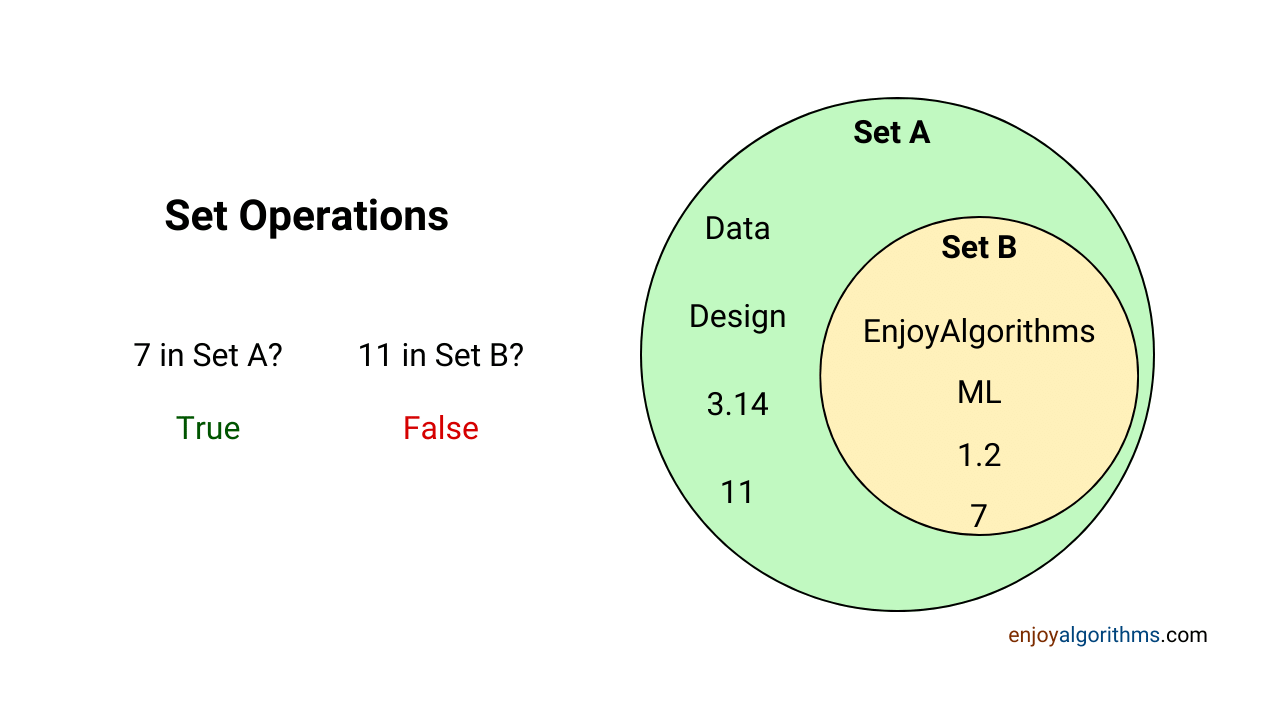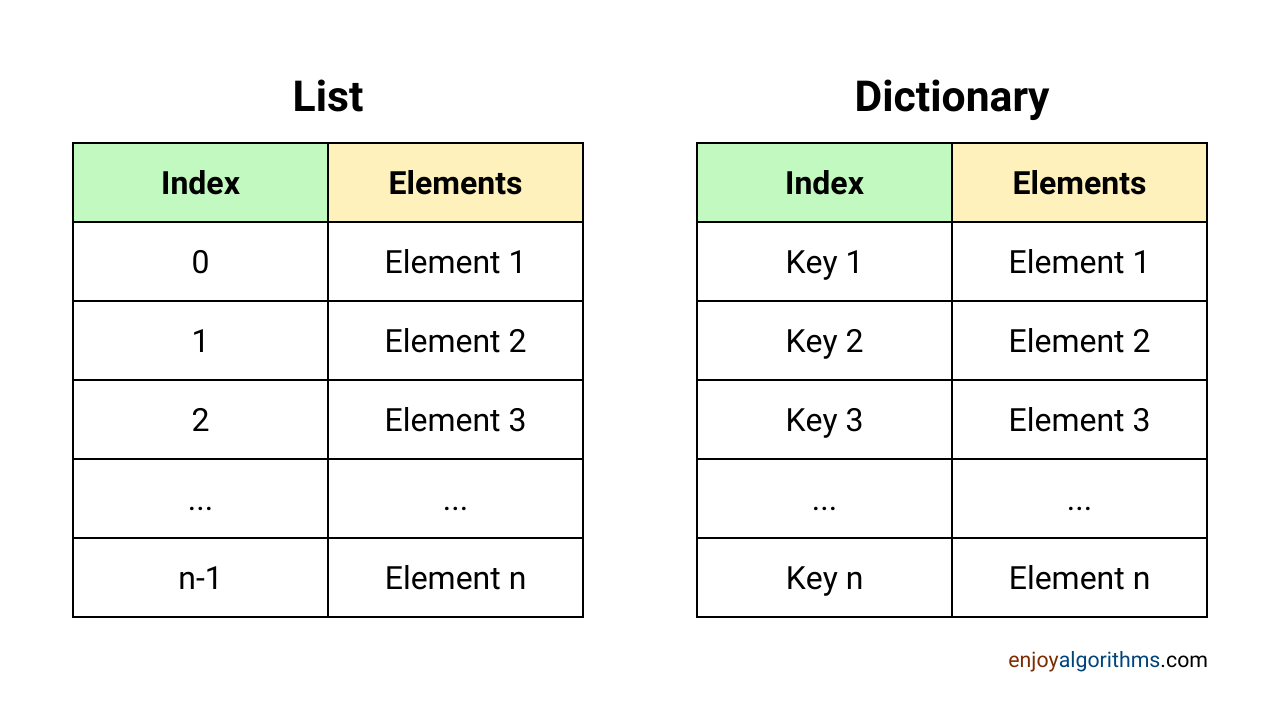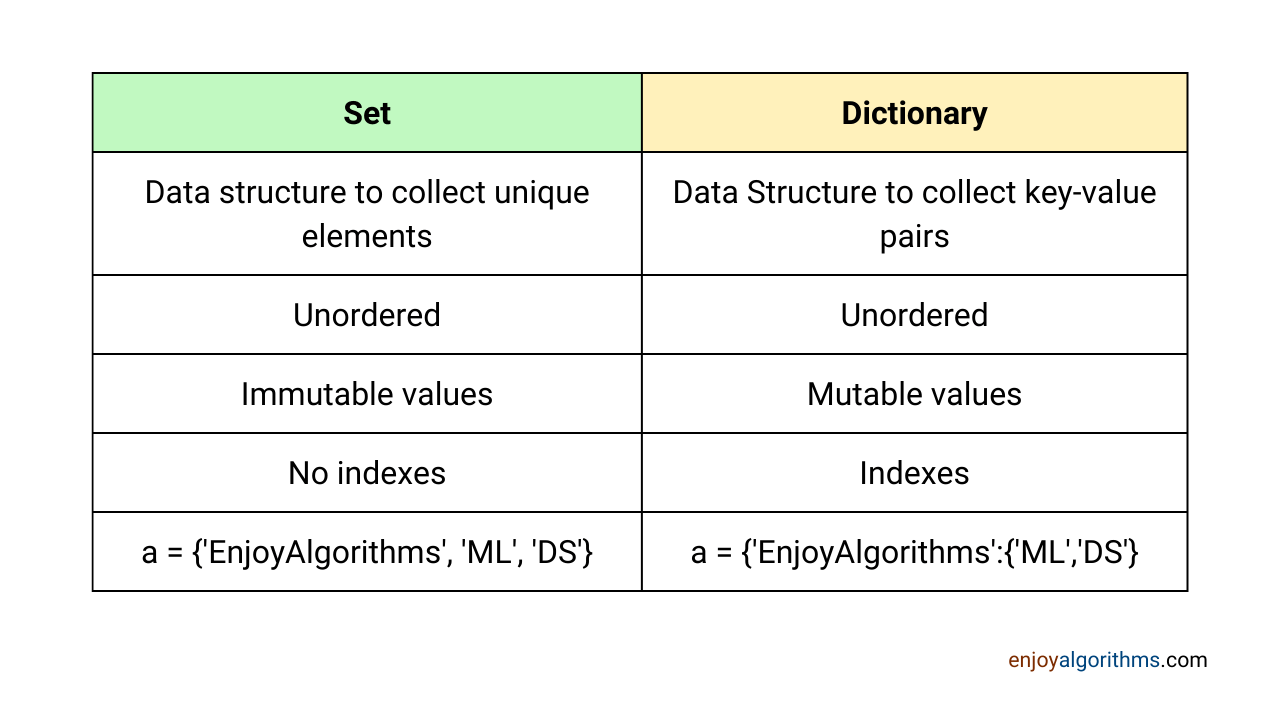# Pyhton Data Structures: Sets and Dictionaries

In Machine Learning and Data Science fields, we need to store a large amount of data so that our algorithms can access them efficiently. That’s where Data Structures come into the picture. In our previous article, we learned about two important data structures in Python used in ML and Data Science, Tuples and Lists. This article will cover other data structures frequently used in AI applications: Sets and Dictionaries.

#### Key takeaways from the blog

After reading this blog, we will be able to understand the following things:

• Concept of sets in Python.
• Operations performed on sets.
• How to convert lists into sets?
• The idea of dictionaries in Python.
• Operations performed on dictionaries.
• Comparison between sets and dictionaries.

### Sets in Python

Sets are data structures used to store multiple data types together. Because of this property, they are also known as compound data types in Python. Unlike lists and tuples, sets are unordered, which means they do not maintain the position or index of the elements they contain.

• Every element in a set is unique, and duplicate values cannot be stored.
• Sets are defined using curly brackets.
``````>>> a = {'EnjoyAlgorithms', 1.2, 7, 7}

>>> a
{'EnjoyAlgorithms', 1.2, 7}

>>> type(a)
<class 'set'>

>>> a = {'EnjoyAlgorithms', 1.2, (7, 7)}
>>> a
{'EnjoyAlgorithms', 1.2, (7, 7)}``````

When we print the created set, we will notice that duplicates are automatically removed. However, when we include duplicates as a tuple, they will be stored in the same form without removal. This is because a tuple such as (7, 7) is treated as a single entity. But we can not store two (7,7).

#### Converting lists or tuples into sets

We can convert a list or tuple data type into a set using the built-in ‘set()’ function. Converting one data type to another is known as type casting. For example, you can use set(mylist) to convert the list mylist into a set. This will automatically remove duplicates in the original list and give you a ‘set’ data type in return.

Let’s understand this using example:

``````>>> a = ('a','b','c', 'd')

>>> set(a)
{'c', 'd', 'a', 'b'}

>>> a = ['a', 'b', 'c', 'd']

>>> set(a)
{'c', 'd', 'a', 'b'}

>>> a = ['a', 'a', 'a', 'b']

>>> set(a)
{'a', 'b'}``````

An exciting thing to note here: Lists and Tuples store elements in a specific order, so the order in which elements are stored matters. However, sets do not store any information about the position of elements, so after conversion, the order of elements in the original list or tuple is lost. Additionally, only the unique elements are retained when we convert a list (with duplicates) into a set.

This property can be helpful in ML applications. For example, we have a huge dataset and want to see how many output categories are present. We can convert the output list into sets which will remove duplicates and give unique types.

#### Nesting in Sets

A set can store other sets as elements, creating a hierarchical structure. For example, a set called “animals” can contain several sets of different types of animals, such as “mammals”, “birds”, and “reptiles”. Depending on the specific use case, these nested sets can then contain other sets or individual elements. This nestedness in sets allows them to store more complex and organized data structures, but it also makes data access difficult.

``a = {'EnjoyAlgorithms', 1.2, (7, 7), {7,7}}``

#### How to access elements from a set in Python?

As we discussed, sets do not contain information about positions, so we can not access elements using the index values. But we can check whether a particular element is present in a given set. For example:

``````>>> a = {'a', 'b', 'c', 'd', 'e', 'f', 'g'}

>>> 'e' in a
True``````

#### How to add elements in a set?

Single Element: We can add elements in a given set using the “.add” operation for single elements. For example:

``````>>> a = {'a', 'b', 'c', 'd'}

>>> a
{'e', 'a', 'b', 'd', 'c'}

>>> a
{'c', 'a', 'b', 'd'}``````

Multiple Elements: We can add multiple elements in a given set using the “.update” operation. For example:

``````>>> a
{'c', 'a', 'b', 'd'}

>>> b = ['e', 'f', 'g']

# Now we want to add b into a
>>> a.update(b)

>>> a
{'c', 'g', 'd', 'a', 'f', 'b', 'e'}``````

Now we know how to add elements inside a set. Let’s also learn about removing elements from a given set.

#### How to remove elements from a set?

To remove an element from a set, we can use either the “.remove()” or “.discard()” operation. It’s important to note that removing multiple elements at once is impossible, so if we need to remove multiple elements, we need to do it one at a time.

``````>>> a = {'EnjoyAlgorithms', 1.2, 7, 'ML'}
>>> a.remove(1.2)

>>> a
{'EnjoyAlgorithms', 'ML', 7}

>>> a
{'EnjoyAlgorithms', 7}``````

#### How to find the length of a set?

We can use the len() function that we saw in the case of lists and tuples to find the length of a given set in Python.

``````>>> a = {'EnjoyAlgorithms', 1.2, 7, 'ML'}
>>> len(a)
4``````

We have seen multiple things about Sets, but one of the key things that make sets unique in Python is the support of mathematical operations. Let’s quickly see how these Sets can be used to find unions, intersections, or other mathematical operations.

#### Mathematical Operations of Sets in Python

If we are familiar with the sets in mathematics, we might know that sets can be defined using a Venn diagram or circular representation. For example, in the diagram below, there are two sets, A and B. Set B is a subpart of Set A, so all the elements present in B are, by default, elements of Set A.There are a lot of important mathematical operations that can be done using Sets. So let’s list some common and very famous operations.

#### Union of Sets

Union is an operation used to form a new set out of all available sets containing all the unique elements inside the individual sets. In simple terms, a union consists of all elements from all the separate sets. We can use the (|) operator in Python to calculate the union. For example:

``````>>> a = {'EnjoyAlgorithms', 1.2, 7, 'ML'}
>>> b = {'Python', 'C++', 'Java', 'EnjoyAlgorithms'}

>>> a|b
{'ML', 1.2, 7, 'C++', 'Python', 'Java', 'EnjoyAlgorithms'}``````

We can also use the “.union()” operation to find the union. The general syntax would be set.union(set1, set2…). For example:

``````>>> a = {'EnjoyAlgorithms', 1.2, 7, 'ML'}
>>> b = {'Python', 'C++', 'Java', 'EnjoyAlgorithms'}

>>> c = {'SS', 'OOPs'}
>>> d = a.union(b,c)

>>> d
{'ML', 1.2, 7, 'C++', 'Python', 'Java', 'SS', 'EnjoyAlgorithms', 'OOPs'}``````

Please note that two individual sets can contain similar elements, but only one element will be present in the resulting set when we do the union operation.

#### Intersection

Using intersection operation, we can form a new set containing common elements from all the sets. We can use Python’s (&) ampersand operator to find the intersection of sets. For example:

``````>>> a = {'EnjoyAlgorithms', 1.2, 7, 'ML'}
>>> b = {'Python', 'C++', 'Java', 'EnjoyAlgorithms'}

>>> a&b
{'EnjoyAlgorithms'}

>>> a.intersection(b)
{'EnjoyAlgorithms'}

>>> c = {'a', 'b'}

>>> a.intersection(b, c)
set()``````

As we have only one element in common between sets a and b, “EnjoyAlgorithms”, hence the output is “EnjoyAlgorithms”. We can also use the “.intersection()” operator to find the intersection. The general syntax would be set.intersection(set1, set2 … etc). If there are no common elements, it will produce an empty set, as shown in the example above.

#### Set Difference

We can also find the difference between the two sets using the (-) operator in Python. If we subtract sets A and B using A-B, the resultant would be a new set with elements unique to set A. For example:

``````>>> a = {'EnjoyAlgorithms', 1.2, 'ML', 7}
>>> b = {'Python', 'C++', 'Java', 'EnjoyAlgorithms'}

>>> a-b
{'ML', 1.2, 7}``````

#### Checking if one set is a subset of other

We can also check whether a given set is a subset of another set. A set is a subset of another set if all the elements present in the former set can be found in the latter set. For example:

``````>>> a = {'ML', 'DataScience', 'RL', 'DL', 'NN'}
>>> b = {'DL', 'NN'}

>>> b.issubset(a)
True``````

#### Copy method in set

It’s important to note in sets that if we assign the value of one set to a new set and then perform operations on the newer set, those operations will also be automatically performed on the original set. For example:

``````>>> a = {'ML', 'DataScience', 'RL', 'DL', 'NN'}
>>> b = a

>>> b.remove('RL')

>>> b
{'ML', 'NN', 'DataScience', 'DL'}

>>> a
{'ML', 'NN', 'DataScience', 'DL'}``````

But we might be thinking about how to perform this assignment as it’s an important feature. So we need to use the “.copy()” operator to copy the original set and perform operations.

``````>>> a = {'ML', 'DataScience', 'RL', 'DL', 'NN'}
>>> b = a.copy()

>>> b.remove('RL')

>>> b
{'ML', 'NN', 'DataScience', 'DL'}

>>> a
{'ML', 'NN', 'DataScience', 'DL', 'RL'}``````

#### Miscellaneous

``````>>> a = {1, 2, 3, 4, 5}

>>> min_a = min(a)
1

>>> max_a = max(a)
5

>>> sum_a = sum(a)
15

>>> b = {3, 4, 5, 6, 7}

>>> a.intersection_update(b)

>>> a
{3, 4, 5}
>>> a = {1, 2, 3, 4, 5}

>>> a.difference_update(b)
>>> a
{1, 2}``````

#### Use of Sets Data Structure in Machine Learning

Sets are seen infrequently while building ML applications, but it has some key applications.

• Sets are used to find the unique number of categories in the output feature; for example {‘dog’, ‘cat’, and ‘apple’} are three unique categories.
• Sets are used to form the hyperparameter sets. Hyperparameters are some hard-coded values present in ML codes. We try to find the best hyperparameter, and for that, we try out multiple unique values that are stored in sets.

That’s enough for the basic understanding of sets. Let’s learn about our second data structure for this blog, i.e., Dictionaries.

### Dictionaries in Python

A dictionary is another data structure in Python that stores multiple data types in a ‘key and value’ pair. If we remember lists, we saw the integer indexes as addresses for the various elements present in the list. In the same line, we have dictionaries, but instead of integer indexes, here we have strings that point to the list.To create a dictionary, we use curly brackets; likewise, we did in sets. But here, we place Keys followed by a colon and then the corresponding values. The keys must be immutable and unique, which means we can not make two keys with the same name, and once made, we can not change the key.

Also, the values for any key can be immutable, mutable, or even duplicates. We can also store lists, tuples, or sets as the values corresponding to a particular key inside a Dictionary, and the pair of keys and values would be separated by a comma (,). For example:

``````>>> a = {'key1':[1,2,3], 'key2':'EnjoyAlgorithms', 'key3':7, 'key4':{1,3,5}}

>>> type(a)
<class 'dict'>

>>> alpha = {1.2:1.2, 7:7, "This is awesome": 11}

>>> type(alpha)
<class 'dict'>``````

Please note that these keys can be strings, integers, or floats. Let’s discuss some essential operations that can be performed on dictionaries.

#### How to access elements from a dictionary in Python?

We can extract an element from a dictionary using the reference for the corresponding key in square brackets. There is one another .get() operator to do the same. For example:

``````>>> a = {'key1':[1,2,3], 'key2':'EnjoyAlgorithms', 'key3':7, 'key4':{1,3,5}}

>>> a['key2']
'EnjoyAlgorithms'

>>> a.get('key2')
'EnjoyAlgorithms'``````

#### How to add elements in a dictionary in Python?

We can add a new “key-value” pair with an assignment operation, given_dict[key] = value. For example:

``````>>> a = {'key1':[1,2,3], 'key2':'EnjoyAlgorithms', 'key3':7, 'key4':{1,3,5}}
>>> a['key5'] = (1,2,4)

>>> a
{'key1': [1, 2, 3], 'key2': 'EnjoyAlgorithms', 'key3': 7, 'key4': {1, 3, 5}, 'key5': (1, 2, 4)}``````

Please note that no ‘key5’ was earlier, but it appeared after the assignment. If there is already a key with the name ‘key5’, the above operation will update the values for ‘key5’.

#### How to change the value of a key in a Python dictionary?

We can assign the new value to the corresponding key. For example:

``````>>> a = {'key1':[1,2,3], 'key2':'EnjoyAlgorithms', 'key3':7, 'key4':{1,3,5}}

>>> a['key4'] = 'ML'

>>> a
{'key1': [1, 2, 3], 'key2': 'EnjoyAlgorithms', 'key3': 7, 'key4': 'ML'}``````

How can we change any key in a dictionary?

As we said, keys are immutable; hence we can not change any key in a given dictionary. For that, we need to add a new key with a new name containing the same values as the older key. Later we can delete the older key.

#### Removing a key or element from a python dictionary

We can delete a particular key from a given dictionary using the del operation. For example:

``````>>> a = {'key1':[1,2,3], 'key2':'EnjoyAlgorithms', 'key3':7, 'key4':{1,3,5}}
>>> del a['key4']

>>> a
{'key1': [1, 2, 3], 'key2': 'EnjoyAlgorithms', 'key3': 7}``````

#### Get all keys and all values of a python dictionary

We can get a list of all the keys and values separately by using .keys() and .values() operators, respectively. For example:

``````>>> a = {'key1':[1,2,3], 'key2':'EnjoyAlgorithms', 'key3':7, 'key4':{1,3,5}}

>>> a.keys()
dict_keys(['key1', 'key2', 'key3', 'key4'])

>>> a.values()
dict_values([[1, 2, 3], 'EnjoyAlgorithms', 7, {1, 3, 5}])``````

#### ‘fromkeys’ method in a Python Dictionary

The fromkeys() method forms a new dictionary with the default values for all the keys mentioned. If we do not define the default values, all values will be assigned to None.

``````>>> a = dict.fromkeys([7,11], 'EnjoyAlgorithms')
>>> a
{7: 'EnjoyAlgorithms', 11: 'EnjoyAlgorithms'}

>>> a = dict.fromkeys([7,11])
>>> a
{7: None, 11: None}``````

#### Nesting of python dictionaries

We can store a new dictionary as a value inside a key. For example:

``````>>> a = {'key1':[1,2,3], 'key2':{'ML':'EnjoyAlgorithms'}}

>>> type(a['key1'])
<class 'list'>

>>> type(a['key2'])
<class 'dict'>``````

#### Use of Dictionaries in Machine Learning and Data Science

Some of the common areas where we can find a dictionary data structure in ML and Data Science are:

1. ML developers need to save a trained model, and generally, it is getting saved in the dictionary format.
2. We can easily find online datasets in a dictionary format to train ML models and perform data science operations.
3. Famous object-detection models like Yolov5 use datasets in the dictionary format.

### Comparison of sets and dictionaries

• Sets can contain only unique values, while dictionaries can contain duplicate values. Dictionaries can not have duplicate keys but can contain identical values.
• Sets and dictionaries both are unordered data structures. In lists and tuples, we can order elements as per their index values, but in sets and dictionaries, these index values are either missing or present as string data types.
• Sets do not have index values, but dictionaries have string indices known as keys.
• Sets are immutable, while values inside a dictionary are mutable in nature.That’s all for understanding the basics of sets and dictionaries.

### Conclusion

In this article, we learned about two important data structures frequently used in Machine learning and data science domains, Sets and Dictionaries. We looked into various operations that can be performed on these data structures and how they store elements. This blog summarized all the important data structures in Python for ML and data science domains. We hope you enjoyed the article.

N﻿ext Blog: Conditions and Branching in Python

Enjoy learning!

☆ 16-Week Live DSA Course
☆ 10-Week Live DSA Course